# Boat

A force of 300 kg (3000 N) is required to pull a boat up a ramp inclined at 14° with horizontal.

How much does the boat weight?

Correct result:

m =  1240.07 kg

#### Solution: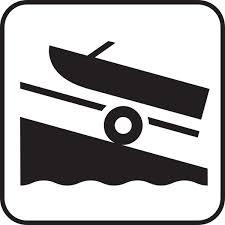We would be pleased if you find an error in the word problem, spelling mistakes, or inaccuracies and send it to us. Thank you!Tips to related online calculators

#### You need to know the following knowledge to solve this word math problem:

We encourage you to watch this tutorial video on this math problem:

## Next similar math problems:

• Inclined plane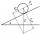On the inclined plane with an angle of inclination of 30 ° we will put body (fixed point) with mass 2 kg. Determine the acceleration of the body motion on an inclined plane.
• Inclined plane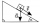The body stays on an inclined plane and exerts a compressive force of 70N on it. Find the angle between the inclined plane and the horizontal if a gravitational force of 100N acts on the body.
• Inclined plane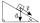1. How much work W we have to do to pull a body weighing 200 kg along an inclined plane with a length of 4 m to a total height of 1.5 m. 2. Find the force we need to exert to do this if we neglect frictional resistance. 3. Find the force we would need if
• ClimbRoad has climbing 1:27. How big is a angle corresponds to this climbing?
• Elevation anglesFrom the endpoints of the base 240 m long and inclined at an angle of 18° 15 ', the top of the mountain can be seen at elevation angles of 43° and 51°. How high is the mountain?Mast has 13 m long shadow on a slope rising from the mast foot in the direction of the shadow angle at angle 15°. Determine the height of the mast, if the sun above the horizon is at angle 33°. Use the law of sines.
• RhombusABCD is a rhombus, ABD is an equilateral triangle and AC is equal to 4. Find the area of the rhombus.
• CosineCalculate the cosine of the smallest internal angle in a right-angled triangle with cathetus 3 and 8 and with the hypotenuse 8.544.
• ClimbOn the road sign, which informs the climb is 8.7%. Car goes 5 km along this road. What is the height difference that car went?
• An observerAn observer standing west of the tower sees its top at an altitude angle of 45 degrees. After moving 50 meters to the south, he sees its top at an altitude angle of 30 degrees. How tall is the tower?
• Traffic laws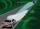Under traffic regulations, car lights can illuminate the road up to a maximum of 30 m. To check the reach of the dipped-beam lights of their car, Peter stopped car at 1.5 m from the wall. The dipped-beam headlights are 60 cm high. At what height on the wa
• Angle in RTDetermine the size of the smallest internal angle of a right triangle whose sides constitutes sizes consecutive members of arithmetic progressions.
• Triangle KLBIt is given equilateral triangle ABC. From point L which is the midpoint of the side BC of the triangle it is drwn perpendicular to the side AB. Intersection of perpendicular and the side AB is point K. How many % of the area of the triangle ABC is area o
• Area and two anglesCalculate the size of all sides and internal angles of a triangle ABC, if it is given by area S = 501.9; and two internal angles α = 15°28' and β = 45°.
• TowerHow many m2 of copper plate should be to replace roof of the tower conical shape with diameter 24 m and the angle at the vertex of the axial section is 144°?
• Two forcesTwo forces with magnitudes of 25 and 30 pounds act on an object at angles of 10° and 100° respectively. Find the direction and magnitude of the resultant force. Round to two decimal places in all intermediate steps and in your final answer.
• Mirror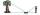How far must Paul place a mirror to see the top of the tower 12 m high? The height of Paul's eyes above the horizontal plane is 160 cm and Paul is from the tower distant 20 m.